# Present Value of 1 Used In Recording a Transaction

Let's use the Present Value (PV) calculation to record an accounting transaction.

On December 31, 2022, Instafix Co. performs a service for MedHealth, Inc. in exchange for a promissory note for \$1,000 that will come due on December 31, 2025. The note does not specify any interest and there is no market for the note. The type of service performed by Instafix Co. is highly customized, meaning the fair market value of the service is not available. Instafix Co.'s accountant must record the transaction on December 31, 2022, keeping in mind the following:

• Instafix Co. cannot record the entire \$1,000 as revenue earned in 2022. Because of the time value of money, part of this amount is interest—interest that will not be earned until the years 2023, 2024, and 2025.

• The \$1,000 is a future value and is not the present value. The cost principle and the revenue recognition principle require that transactions be recorded at their cash value at the time of the transaction. If the cash value is not known, accountants look to the fair market value of the services provided or the fair market value of the property received (in this case the note). Since those amounts are unknown, the accountant estimates the cash equivalent amount by calculating the present value of the note.

To record the cash equivalent amount through a present value calculation, the accountant must estimate the interest rate (i) appropriate for discounting the future amount to the present time. The rate will reflect the length of time before the money will be received as well as the credit worthiness of MedHealth, Inc. Let's assume that the appropriate rate is 10% compounded annually.

The following timeline depicts the information we know, along with the unknown component (PV):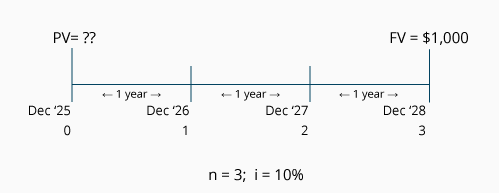As our timeline indicates, the length of time is three years, the interest rate is 10% compounded annually, and the future cash amount is \$1,000. Let's calculate the present value of this single amount by using a PV of 1 table: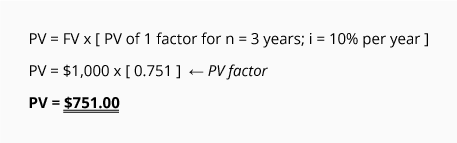Our calculation shows that receiving \$1,000 at the end of three years is the equivalent of receiving approximately \$751.00 today, assuming the time value of money is 10% per year compounded annually.

The cost principle (cash or cash equivalent at the time of the transaction) will be satisfied by recording the amount \$751. The revenue recognition principle will also be satisfied, because only \$751 has been earned (the amount associated with the services performed in December 2022). The \$249 difference between \$1,000 and \$751 is interest that will be earned in the years 2023, 2024, and 2025.

Here is the entry the Instafix Co. accountant records on December 31, 2022: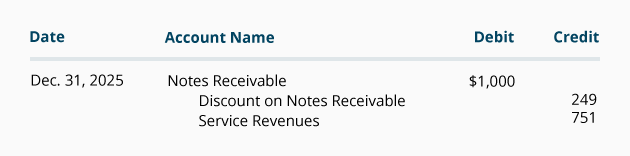In the years 2023 through 2025, the Instafix Co. will record a total of \$249 of interest revenue. If that amount is considered insignificant (with regards to the company's total net income) the company could simply use the straight-line method to record \$83 per year (\$249 ÷ 3 years), as shown in the following journal entry:Over the years 2023 through 2025, the balance in Discount on Notes Receivable will move from a credit balance of \$249 to a balance of zero. (Some accountants would describe this as amortizing the \$249 balance to Interest Revenue over the life of the note.) At the end of 2025 and just prior to collecting the \$1,000 due on the note, the carrying value of the note will be \$1,000 (the \$1,000 balance in Notes Receivable minus the \$0 balance in Discount on Notes Receivable).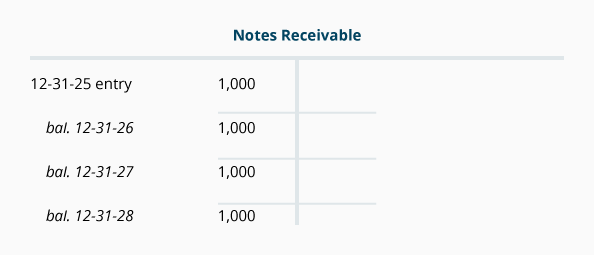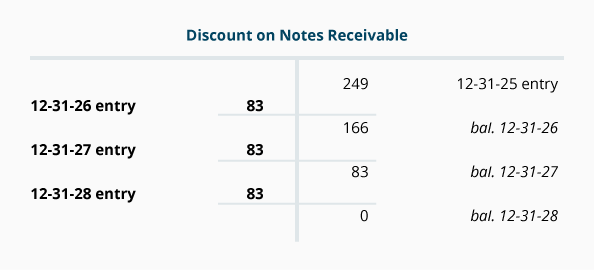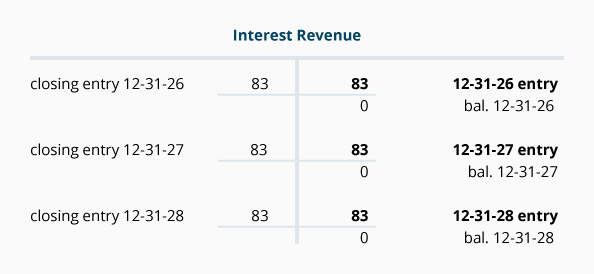Effective Interest Rate Method of Amortizing the Discount

If, however, the \$249 of interest is considered significant, then the amount of interest recorded each year should be determined by the effective interest rate method. Under this method, the amount of interest revenue recorded is directly tied to the balance (or carrying amount) of the note receivable. In other words, over the years, the interest revenue will increase as the note's carrying value increases: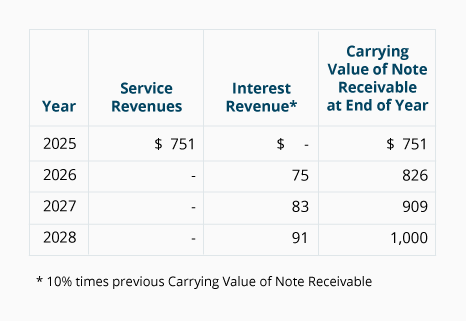Using the effective interest rate method for interest earned, the journal entries to amortize Discount on Notes Receivable to Interest Revenue over the life of the note are as follows: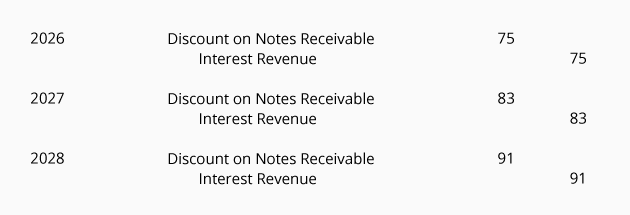After posting these journal entries, the account balances will appear as follows: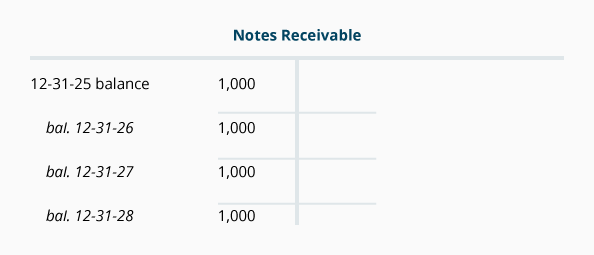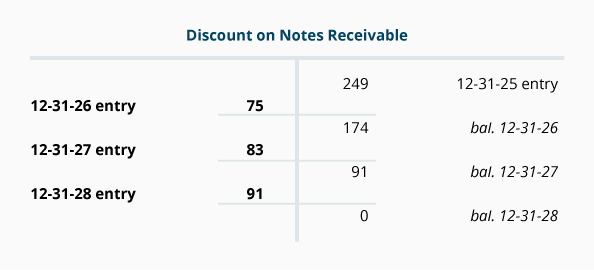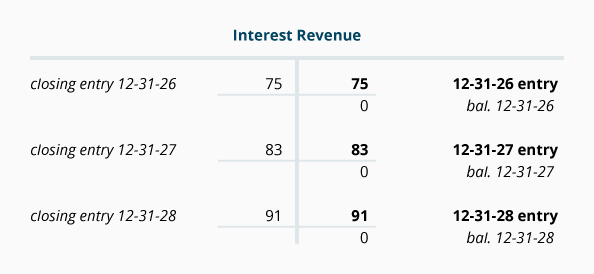The effective interest rate method must be used when the amount of the discount is significant. The reason for requiring this method of amortizing is to exhibit the logical relationship between the carrying value of the note reported on the balance sheet and the interest reported on the income statement.

#### Take Our Practice Quiz

We recommend that you now take our free Practice Quiz for this topic so that you can...

• See what you know
• See what you don't know